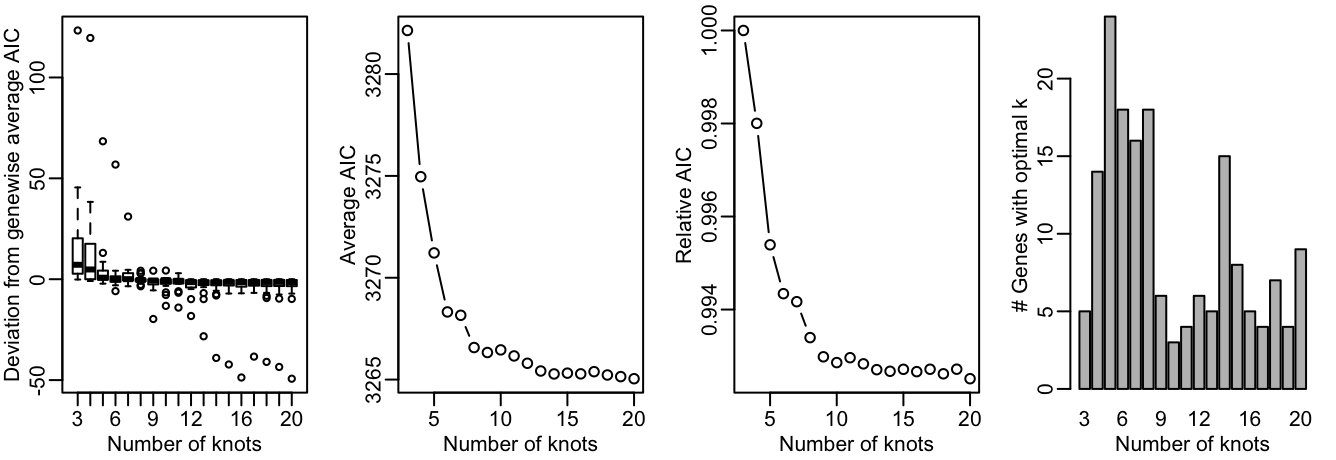tradeSeq is an R package that allows analysis of gene expression along trajectories. While it has been developed and applied to single-cell RNA-sequencing (scRNA-seq) data, its applicability extends beyond that, and also allows the analysis of, e.g., single-cell ATAC-seq data along trajectories or bulk RNA-seq time series datasets. For every gene in the dataset, tradeSeq fits a generalized additive model (GAM) by building on the mgcv R package. It then allows statistical inference on the GAM by assessing contrasts of the parameters of the fitted GAM model, aiding in interpreting complex datasets. All details about the tradeSeq model and statistical tests are described in our preprint (Van den Berge et al. 2019).

In this vignette, we analyze a subset of the data from (Paul et al. 2015). A SingleCellExperiment object of the data has been provided with the tradeSeq package and can be retrieved as shown below. The data and UMAP reduced dimensions were derived from following the Monocle 3 vignette.

In this vignette, we use tradeSeq downstream of slinghsot(Street et al. 2018) but it can be used downstream of any trajectory. In particular, if you want to use tradeSeq downstream of monocle(Qiu et al. 2017) and monocle3(Cao et al. 2019), please refer to our Monocle vignette.

Installation

To install the package, simply run

if(!requireNamespace("BiocManager", quietly = TRUE)) {
install.packages("BiocManager")
}

library(RColorBrewer)
library(SingleCellExperiment)
library(slingshot)

# For reproducibility
RNGversion("3.5.0")
palette(brewer.pal(8, "Dark2"))
counts <- as.matrix(countMatrix)
rm(countMatrix)

Fit negative binomial model

Here we fit the tradeSeq negative binomial generalized additive model (NB-GAM). Please see the fitGAM vignette for an extensive description on how to fit the models, tune its options and modify its output.

We first need to decide on the number of knots. This is done using the evaluateK function. This takes a little time to run so it is not run here.

set.seed(5)
icMat <- evaluateK(counts = counts, sds = crv, k = 3:10,
nGenes = 200, verbose = T)For more explanation on the output from evaluateK, we refer users to the fitGAM vignette. Here, we pick nknots = 6.

We then fit the models by running the fitGAM function. By default, the gene-wise NB-GAM estimates one smoother for every lineage using the negative binomial distribution. Please refer to the fitGAM vignette Additional to add additional covariates to the model, speed up computation or allow for custom normalization, amongst others.

set.seed(7)
pseudotime <- slingPseudotime(crv, na = FALSE)
cellWeights <- slingCurveWeights(crv)
sce <- fitGAM(counts = counts, pseudotime = pseudotime, cellWeights = cellWeights,
nknots = 6, verbose = FALSE)

The model may be hard to fit for some genes, and hence the fitting procedure may not converge for all of the genes in a dataset, especially in datasets with complex patterns and/or many lineages. You can check the convergence of each gene as shown below, where a TRUE value corresponds to a converged model fit, and a FALSE value corresponds to a model that hasn’t been able to converge fully.

##
## TRUE
##  240

Within-lineage comparisons

Association of gene expression with pseudotime

A first exploration of the data analysis may consist of checking whether gene expression is associated with a particular lineage. The statistical test performed here, implemented in the associationTest function, is testing the null hypothesis that all smoother coefficients are equal to each other. This can be interpreted as testing whether the average gene expression is significantly changing along pseudotime.

assoRes <- associationTest(sce)
##        waldStat df       pvalue meanLogFC
## Acin1 130.03742  9 0.000000e+00 0.2912872
## Actb  476.27882  9 0.000000e+00 0.2088096
## Ak2    94.17204  9 2.220446e-16 0.2063961
## Alad  194.07437  9 0.000000e+00 0.3610146
## Alas1 977.87981  9 0.000000e+00 0.4470031
## Aldoa 254.93054  9 0.000000e+00 0.1773934

Discovering progenitor marker genes

In order to discover marker genes of the progenitor or differentiated cell population, researchers may be interested in assessing differential expression between the progenitor cell population (i.e., the starting point of a lineage) with the differentiated cell type population (i.e., the end point of a lineage). The function startVsEndTest uses a Wald test to assess the null hypothesis that the average expression at the starting point of the smoother (progenitor population) is equal to the average expression at the end point of the smoother (differentiated population). The test basically involves a comparison between two smoother coefficients for every lineage. The function startVsEndTest performs a global test across all lineages by default (i.e. it compares the start and end positions for all lineages simultaneously), but you can also assess all lineages separately by setting lineages=TRUE. Below, we adopt an omnibus test across the two lineages.

startRes <- startVsEndTest(sce)

We can visualize the estimated smoothers for the third most significant gene.

oStart <- order(startRes\$waldStat, decreasing = TRUE)
sigGeneStart <- names(sce)[oStart]
plotSmoothers(sce, counts, gene = sigGeneStart)